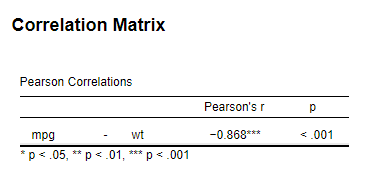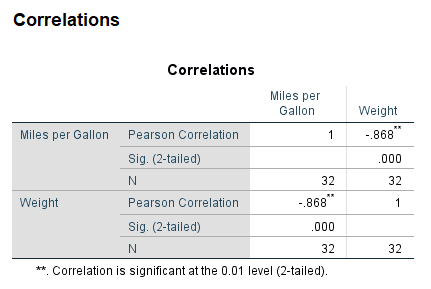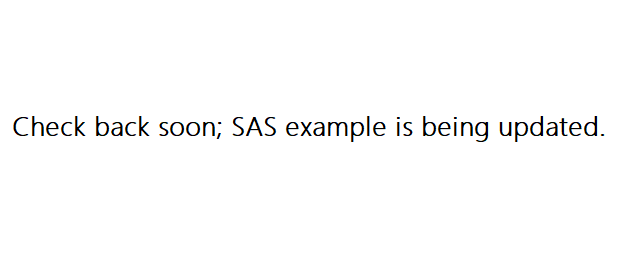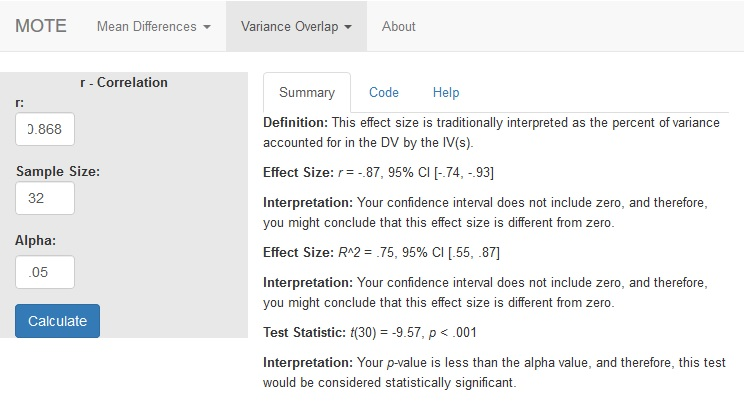# Description

This function displays transformation from r to r2 to calculate the non-central confidence interval for $r^{2}$ using the F distribution.

The formula for t is: $$\frac{r}{\sqrt{ \frac{1-r^2} {(N - 2)}}}$$

# R Function

r.correl(r, n, a)

# Arguments

• r = correlation coefficient
• n = sample size
• a = significance level

# Example

What is the correlation between miles per gallon and car weight? Are lighter cars more fuel-efficient?

This example is derived from the mtcars dataset, provided in R. An amended dataset is available on our GitHub. Example output from JASP, SPSS, and SAS are shown below.

JASPSPSSSAS• r = -0.868
• n = 32
• a = .05

# Function in R:

r.correl(r = -0.868, n = 32, a = 0.05)

# MOTE

## ScreenshotDefinition: This effect size is traditionally interpreted as the percent of variance accounted for in the DV by the IV(s).

## Effect Size:

r = -.87, 95% CI [-.74, -.93]

## Interpretation:

Your confidence interval does not include zero, and therefore, you might conclude that this effect size is different from zero.

## Effect Size:

$R^{2}$ = .75, 95% CI [.55, .87]

## Interpretation:

Your confidence interval does not include zero, and therefore, you might conclude that this effect size is different from zero.

## Test Statistic:

t(30) = -9.57, p < .001

## Interpretation:

Your p-value is less than the alpha value, and therefore, this test would be considered statistically significant.